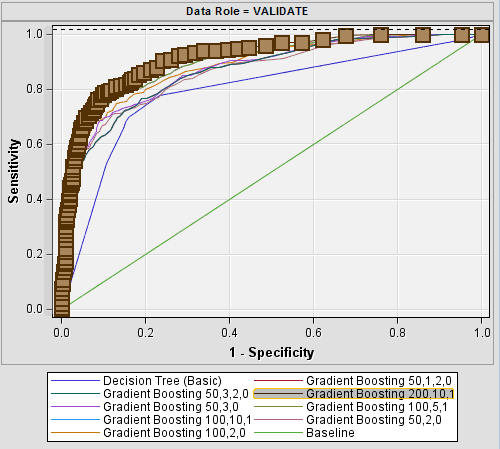Predictive Customer Analytics using Decision Trees with ScikitThe tree-based techniques used for regression or classification have been some of the most prevalent algorithms of machine learning. This consists of putting the space of the forecaster into several strata or simple regions. Furthermore, in order to forecast the variable targeted for a fresh observation, we could make use of both the mean and the mode of the data points used in training in the area where it belongs. As the entire collection of decision-based rules that are making a forecast are denoted by a tree, these styles and approaches to learning are termed as decision tree techniques. As per the type of targeted variable, there are two kinds of decision trees: Classification Tree that has a binary target variable, and the Regression Tree which has a more continuous targeted variable.Figure 1 General Form of Decision Tree

The general form type of tree-based model is shown in Figure 1, and one or more than one decision rules could be attained that help describe the relationship between the inputs (forecasters) and the targets (dependent variable). Moreover, the significance of the forecaster is very apparent and its relations can be seen easily. However, they might lack the predictive accuracy when compared with the finest overseen learning algorithms. Therefore, collective learning methods have been widely presented in order to improve the model accuracy by joining the predictions from many trees. The cost of the collective methods is a little loss in interpretation.

• Simple to Understand: The tree-based graphical depictions are instinctive and easy to interpret, especially for non-technical users.
• Low data cleaning requirement: The process requires much less data cleaning when compared to other modeling methods. It is not influenced by outliers or the missing values to a certain degree.
• Data type is not a constraint: Can be handled by both the numerical and categorical variables.
• Non-Parametric Method: The decision tree is expected to be a non-parametric technique. This means that decision trees do not have assumptions about the space distribution or even the structure of the classifier.

The tree-based predictive analysis models could be applied to the entire customer lifecycle, involving growth of relationship, acquisition, retention, and winning-back. For instance, the decision tree could analyze the spending of customers, their usage and even other behaviors, which could lead to more efficient cross sales, or selling of more products to current customers. This always leads to better profitability per customer and even more fantastic customer experiences. As per the survey of Kaggle 2017, the decision tree models and random forests are ranked as the 2nd and 3rd most regular data analysis techniques.Figure 2 Data analytics methods used at work

The Scikit-learn method offers a variety of both supervised and unsupervised learning algorithms via a reliable interface in Python. This article will show a short introduction of Scikit-learn (which is a machine leaning Python library) to help setup a decision tree and improve the prediction accuracy with ensemble techniques. Some crucial issues in creating a decision tree classifier can be shown as follows.

1. Pre-processing categorical variables

We use Scikit-learning to create the decision tree, we have to firstly state and encode the categorical variables so that the classifier will be able to handle these particular variables.  (Heartwalk is one dataset that can describe the donation actions of the heart walk event that was organized by the American Heart Association).

from sklearn import preprocessing

le_tap = preprocessing.LabelEncoder()heartwalk[‘Tap_life’] = le_tap.fit_transform(heartwalk[‘Tap_life’])

1. Splitting up the data into training and validation sets

# split the full dataset into training(70%) and validating datasets(30%)X = heartwalk.values[:, 1:14]Y = heartwalk.values[:,0]X_train, X_test, y_train, y_test = train_test_split( X, Y, test_size = 0.3, random_state = 100)

1. Setup the decision tree classifier

# simple decision trees. The maximum of tree depth is setting to be 5 that helps control overfitting issue.DT_simple = DecisionTreeClassifier(criterion = “gini”, random_state = 100, max_depth = 5, min_samples_leaf=100)DT_simple.fit(X_train, y_train)

1. Assess the decision trees’ performance

# import the library to evaluate the model performance

from sklearn.metrics import roc_curve

from sklearn.metrics import auc

from sklearn.metrics import accuracy_score

from sklearn.metrics import confusion_matrix

# Get the predicted values and critical indicators for model performance

y_pred = DT_simple.predict(X_test)

print(“Accuracy is {}“.format(accuracy_score(y_test,y_pred)*100))

print(“Confusion matrix is as follows:”)

print(confusion_matrix(y_test, y_pred))

1. Create the visual depiction of decision tree

# Genarate the figure of decision treeimport graphviz from sklearn.externals.six import StringIO  from IPython.display import Image  import pydotplusdot_data = StringIO()tree.export_graphviz(DT_simple, out_file=dot_data,feature_names=heartwalk_model.columns.tolist()[1:14],                 class_names=[‘Non_ZDW’,’ZDW’], filled=True, rounded=True,                special_characters=True)graph = pydotplus.graph_from_Figure 3 Visualizaiton of Decision Tree

1. Gather methods to improve accuracy of prediction

# Using one of ensemble methods, random forest to make prediction. from sklearn.ensemble import RandomForestClassifierRandomTree = RandomForestClassifier()RandomTree.fit(X_train, y_train)y_pred_2 = RandomTree.predict(X_test)# boosting: many many weak classifiers (max_depth=3) refine themselves sequentiallyfrom sklearn.ensemble import GradientBoostingClassifierBoostingTree = GradientBoostingClassifier(n_estimators=100, learning_rate=0.1, max_depth=3, random_state=100)BoostingTree.fit(X_train, y_train)y_pred_3 = BoostingTree.predict(X_test)The major principle of the ensemble model is that a set of weak learners came together to become a strong learner. The ROC analysis shows that the ensemble methods, random forests and even gradient boosting helped to significantly develop the prediction accuracy of a single decision tree.